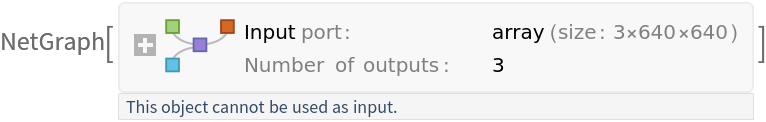# YOLO V8 Pose Trained onMS-COCO Data

Detect and localize human joints in an image

YOLO (You Only Look Once) Version 8 by Ultralytics is the latest version of the YOLO models. Just like its predecessor, YOLO Version 5, YOLO Version 8 is an anchor-free model that was trained with mosaic augmentation. It features the use of new "C2f" blocks, which employ additional dense connections between bottleneck modules. Although YOLO models are historically object detection models, these models perform both object detection and human joint keypoint regression at the same time.

## Training Set Information

• Microsoft COCO, a dataset for image recognition, segmentation, captioning, object detection and keypoint estimation, consisting of more than three hundred thousand images.

## Model Information

•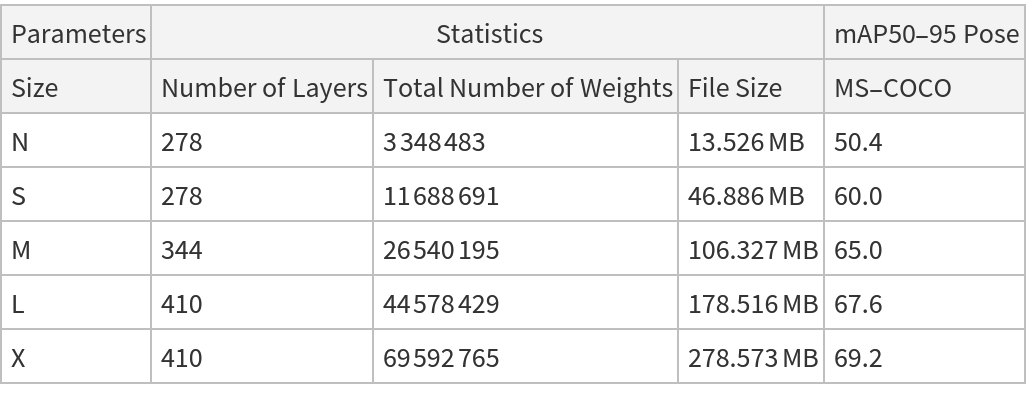## Examples

### Resource retrieval

Get the pre-trained net:

 In:=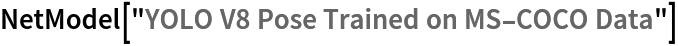Out=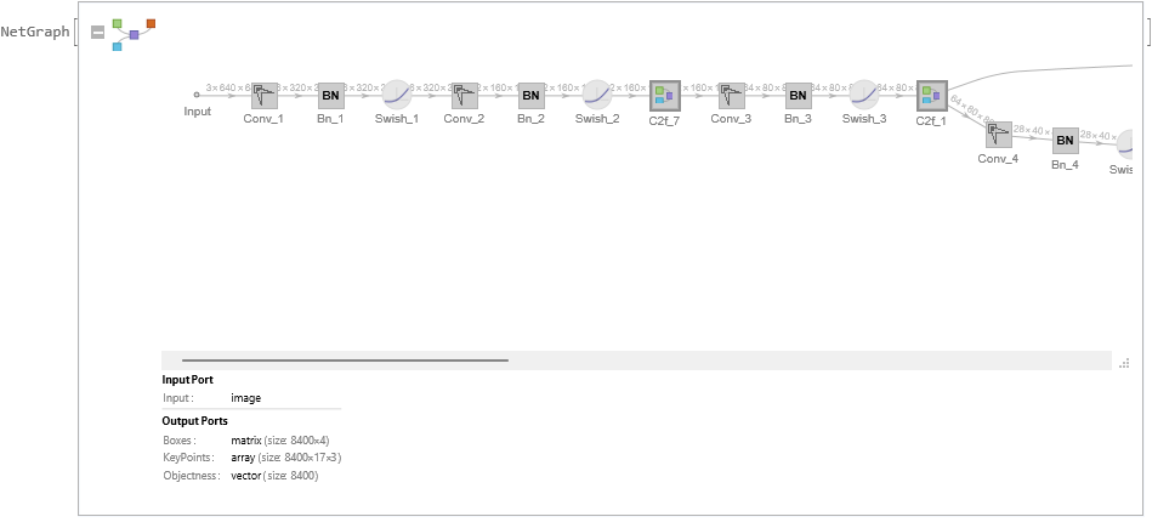### NetModel parameters

This model consists of a family of individual nets, each identified by a specific parameter combination. Inspect the available parameters:

 In:=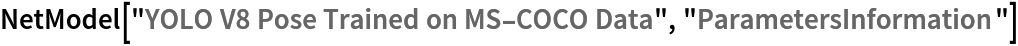Out=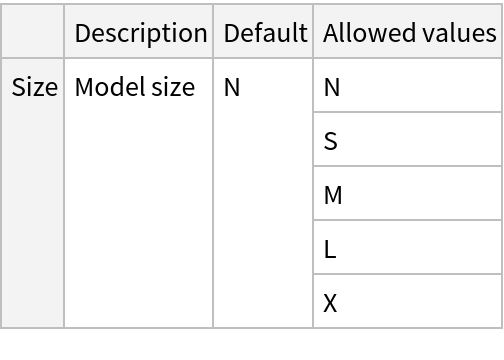Pick a non-default net by specifying the parameters:

 In:=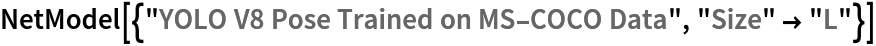Out=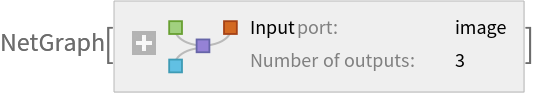Pick a non-default uninitialized net:

 In:=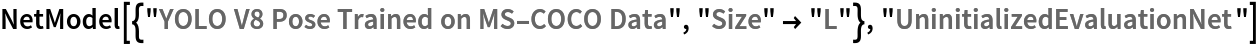Out=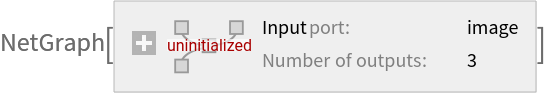### Evaluation function

Write an evaluation function to scale the result to the input image size and suppress the least probable detections:

 In:=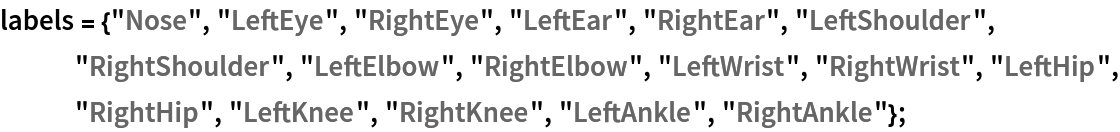In:=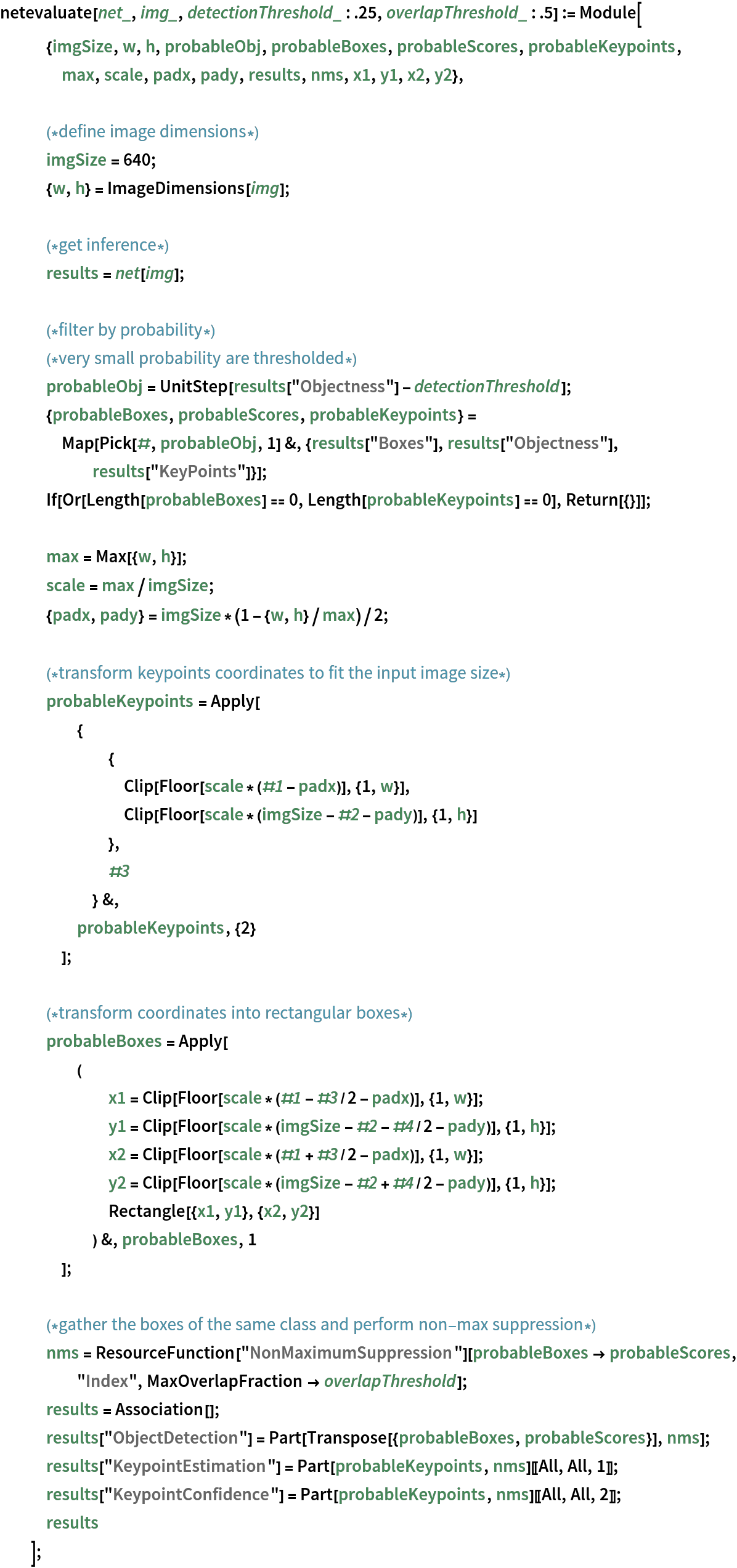### Basic usage

Obtain the detected bounding boxes with their corresponding classes and confidences as well as the locations of human joints for a given image:

 In:=In:=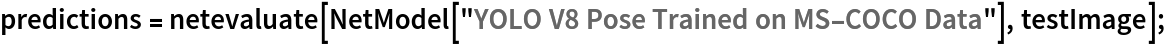Inspect the prediction keys:

 In:=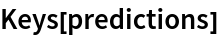Out=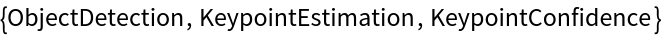The "ObjectDetection" key contains the coordinates of the detected objects as well as their confidences and classes:

 In:=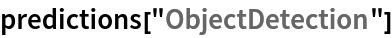Out=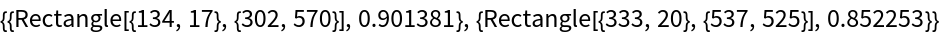The "KeypointEstimation" key contains the locations of the top predicted keypoints:

 In:=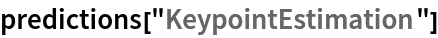Out=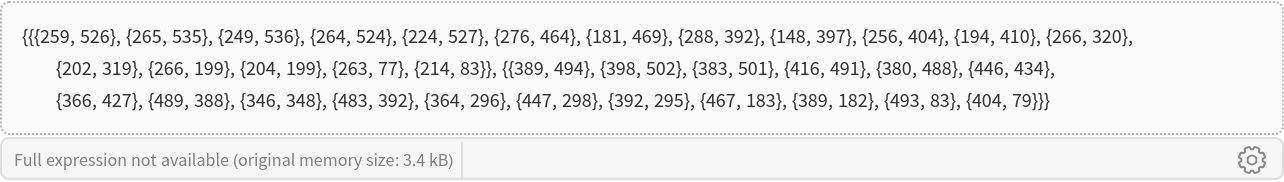The "KeypointConfidence" key contains the confidences for each person’s keypoints:

 In:=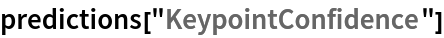Out=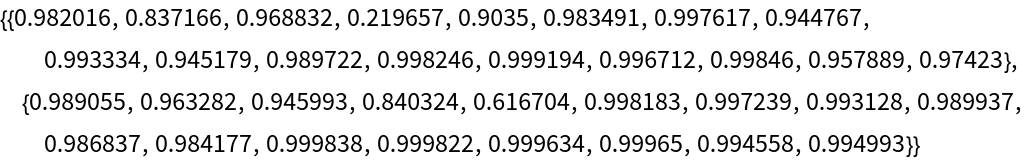Inspect the predicted keypoint locations:

 In:=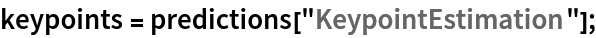Visualize the keypoints:

 In:=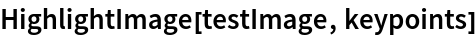Out=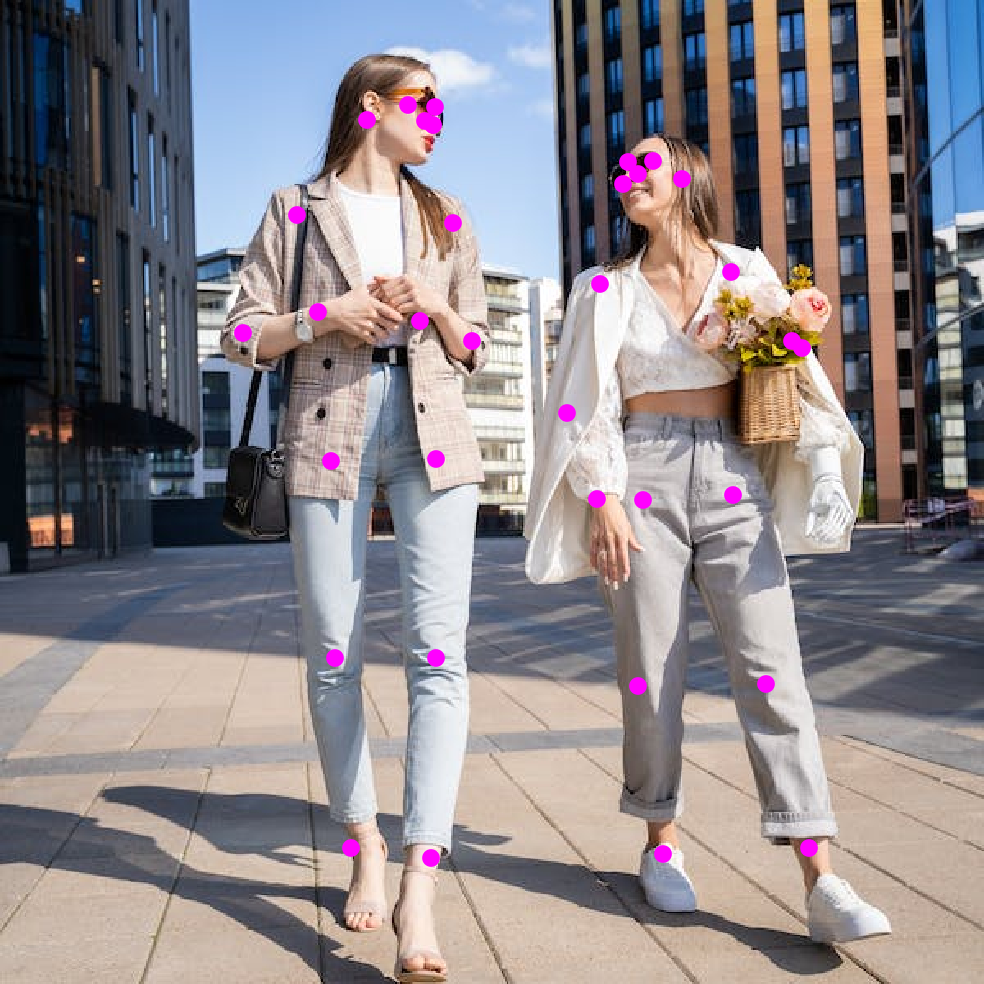Visualize the keypoints grouped by person:

 In:=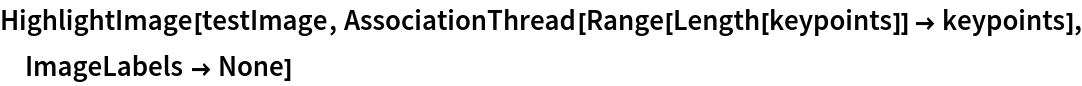Out=Visualize the keypoints grouped by a keypoint type:

 In:=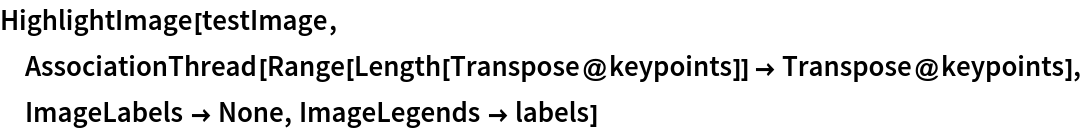Out=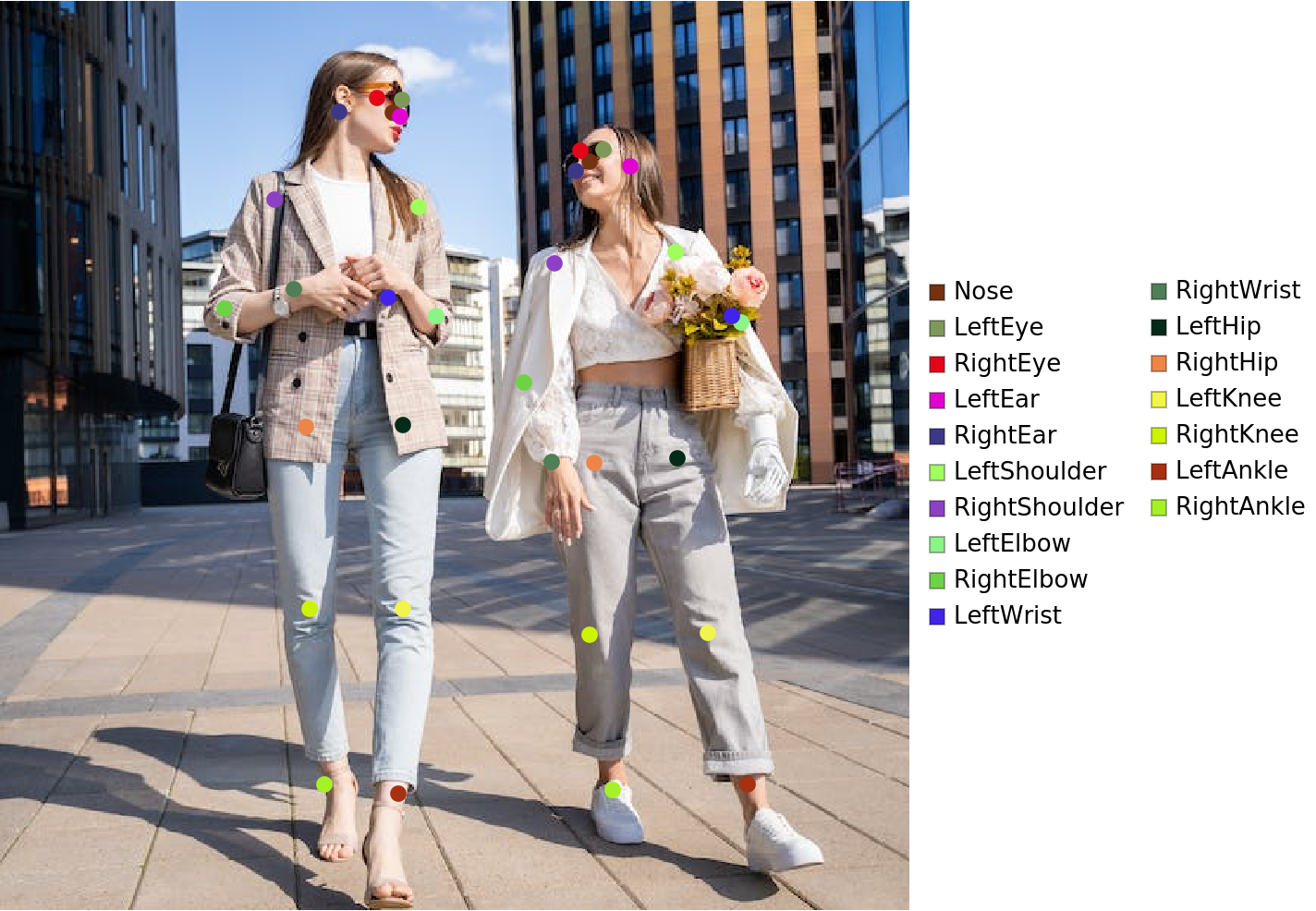Define a function to combine the keypoints into a skeleton shape:

 In:=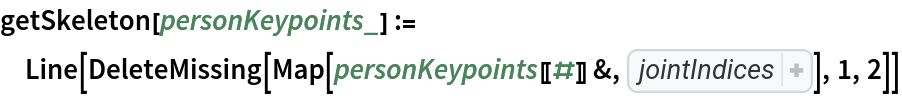Visualize the pose keypoints, object detections and human skeletons:

 In:=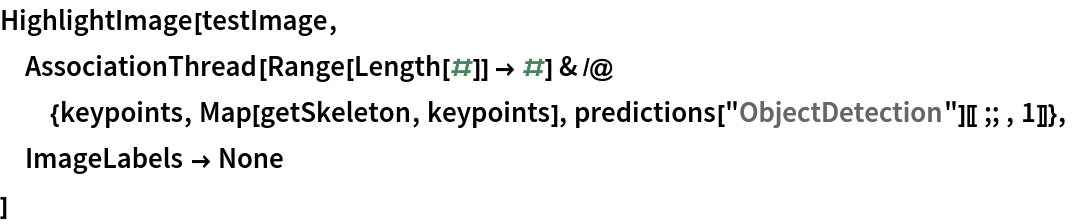Out=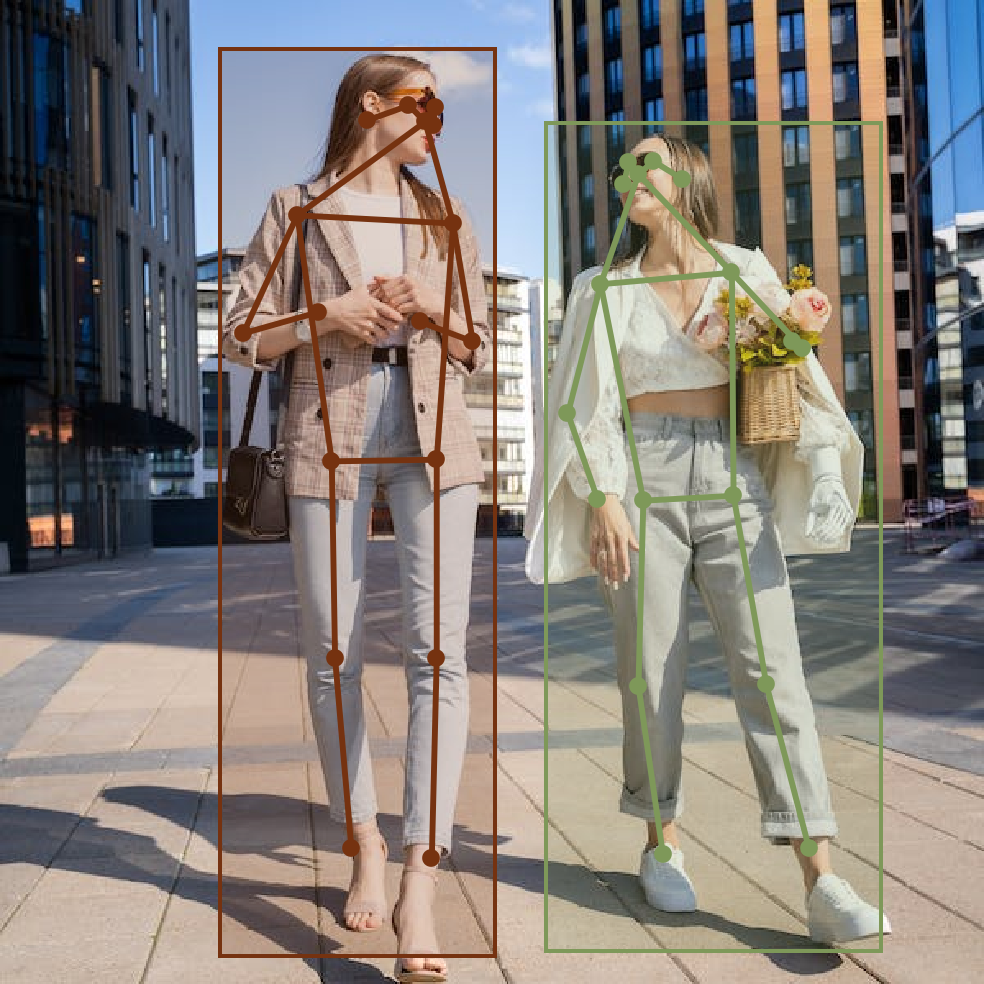### Network result

The network computes eight thousand four hundred bounding boxes, the position of the keypoints with their probabilities and the probability of an object inside the box:

 In:=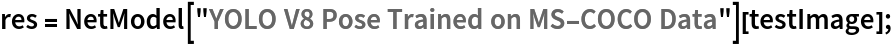In:=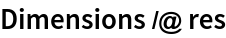Out=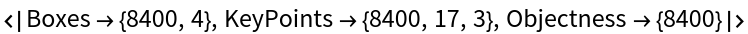Rescale the "KeyPoints" to the coordinates of the input image and visualize them scaled and colored by their probability measures:

 In:=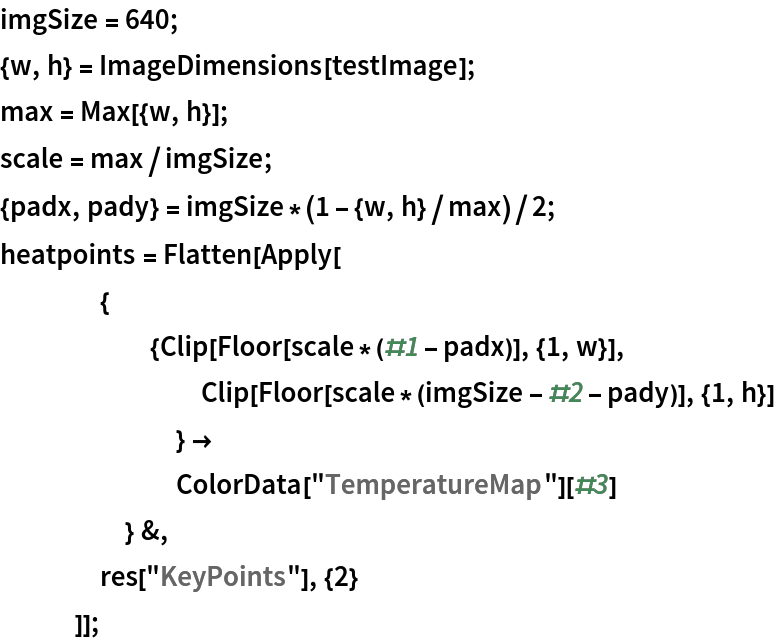In:=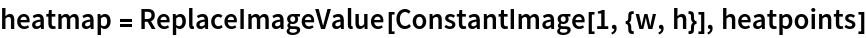Out=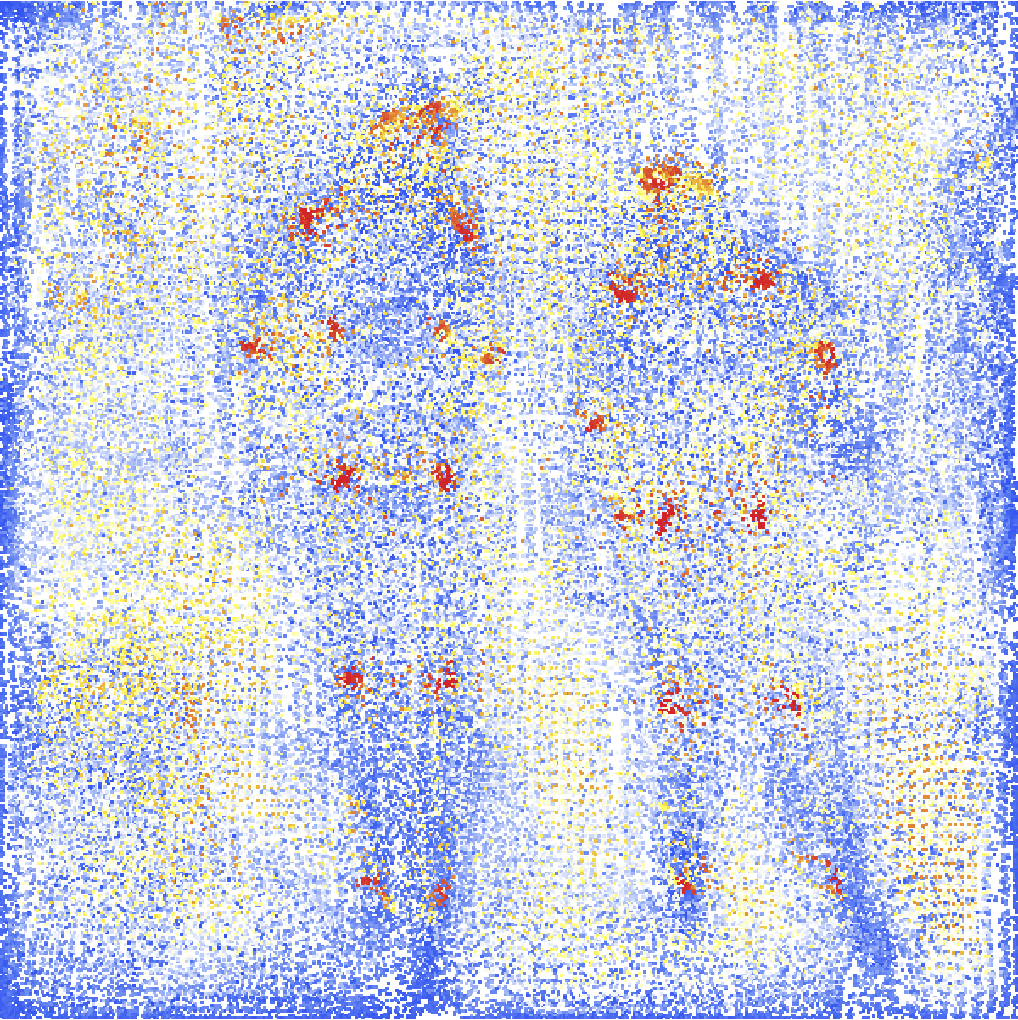Overlay the heat map on the image:

 In:=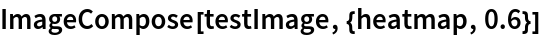Out=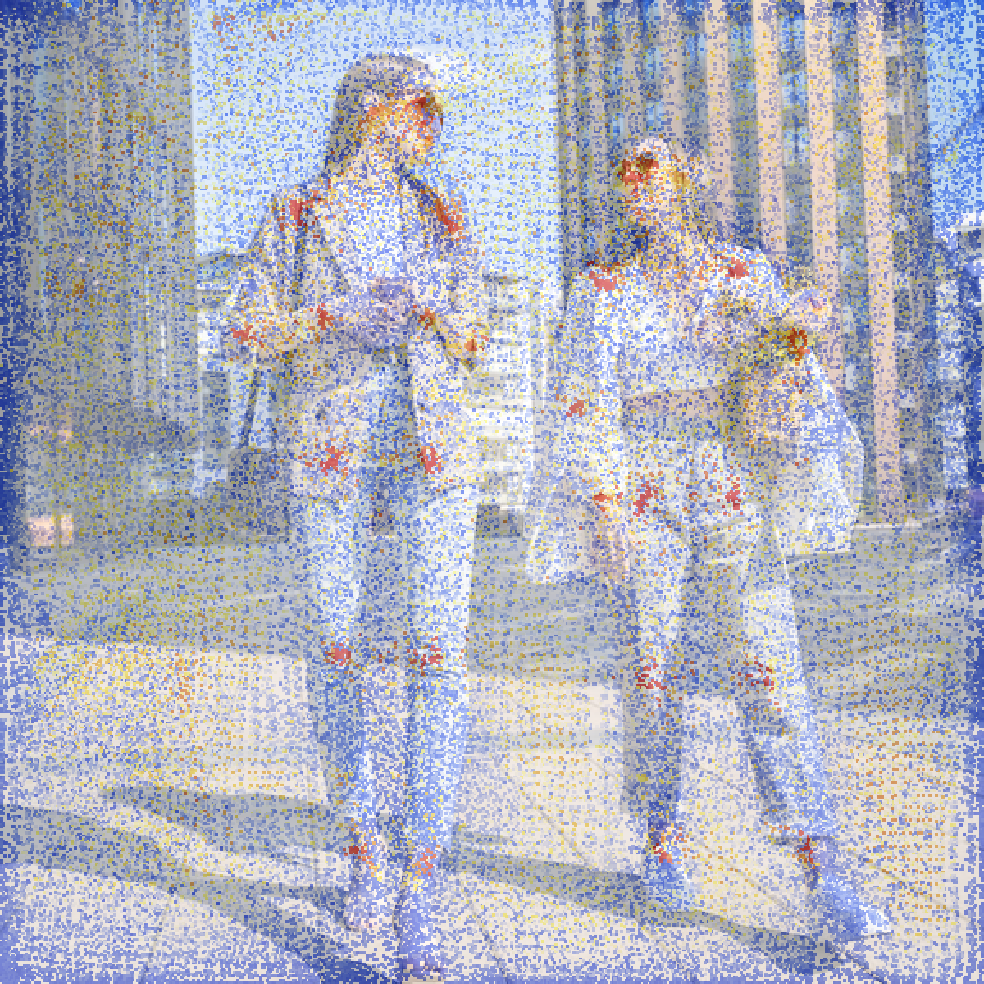Rescale the bounding boxes to the coordinates of the input image and visualize them scaled by their "Objectness" measures:

 In:=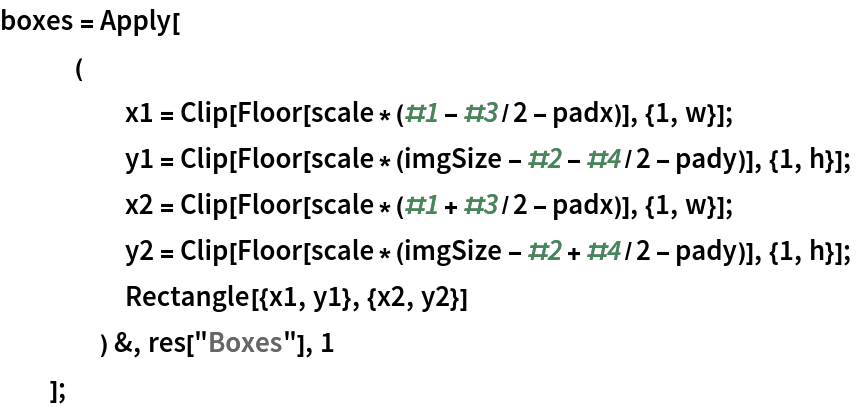In:=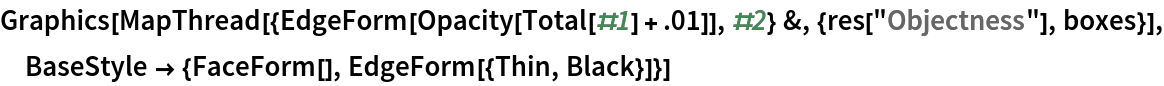Out=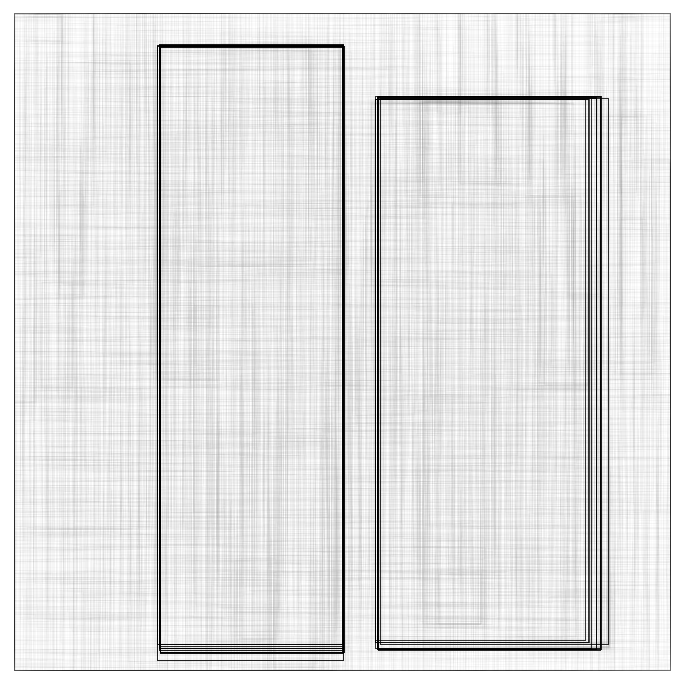Superimpose the predictions on top of the input received by the net:

 In:=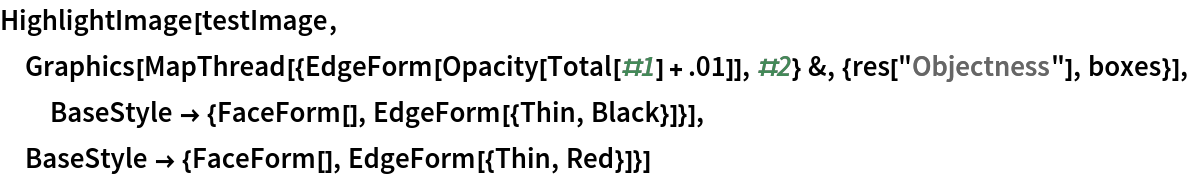Out=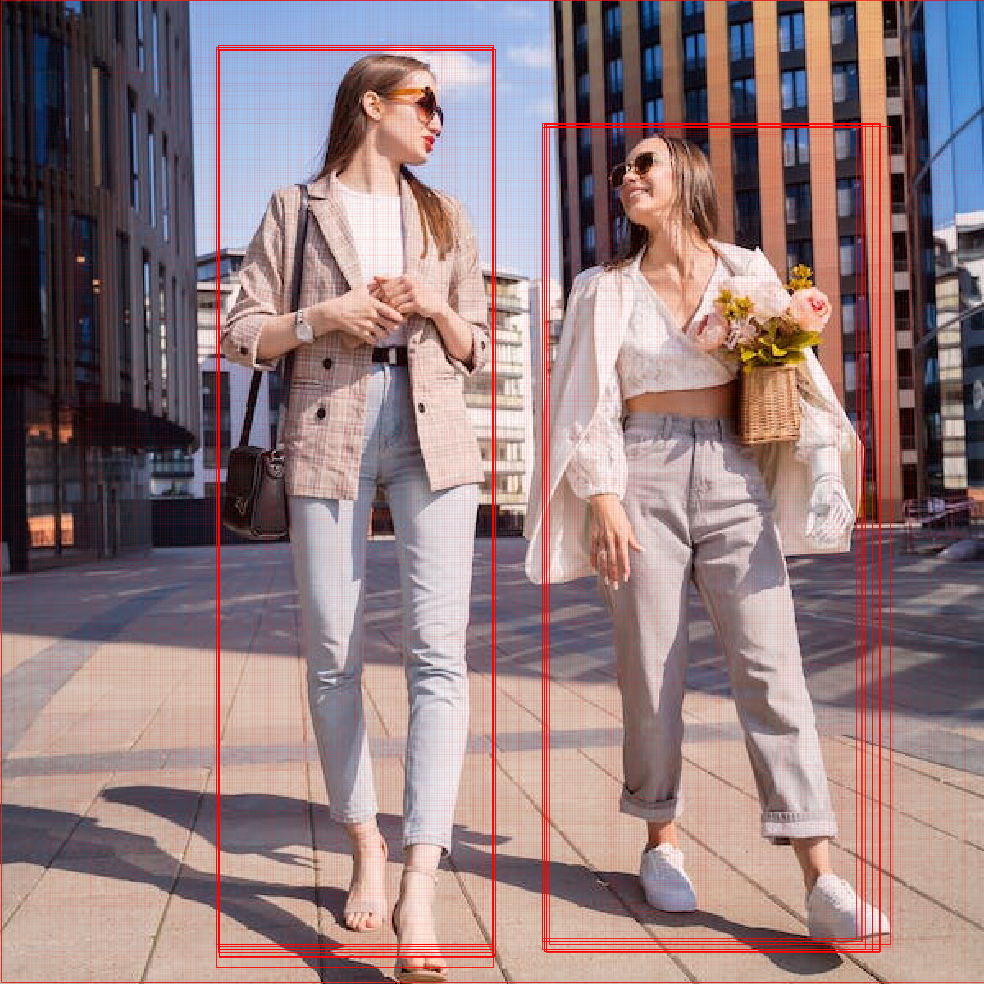### Net information

Inspect the number of parameters of all arrays in the net:

 In:=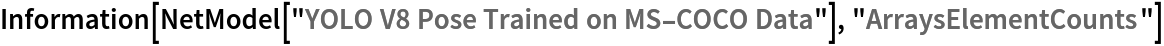Out=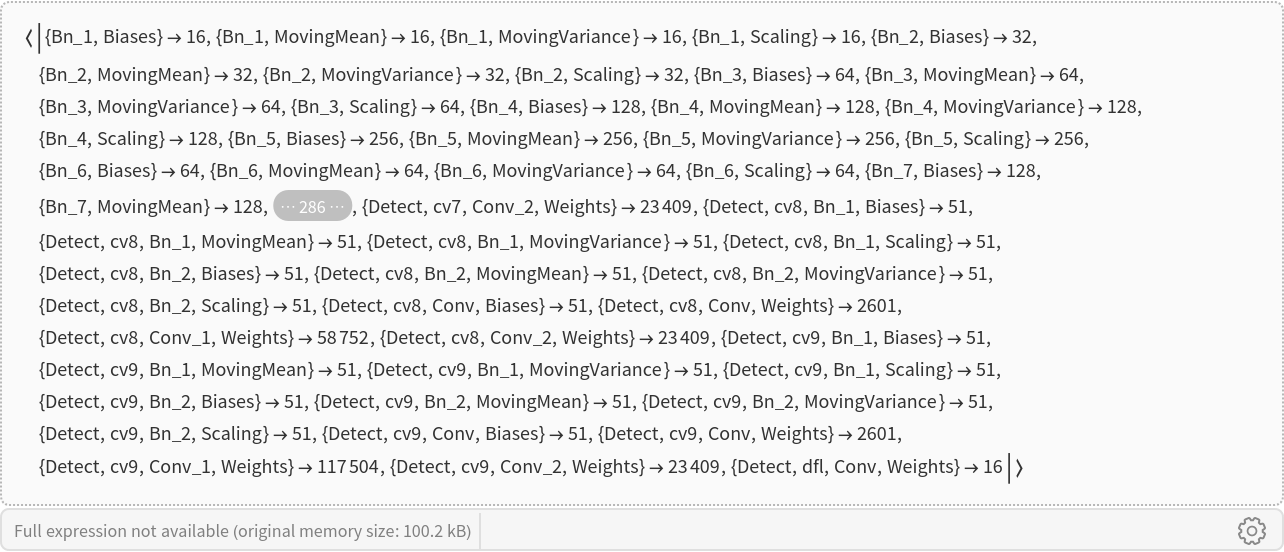Obtain the total number of parameters:

 In:=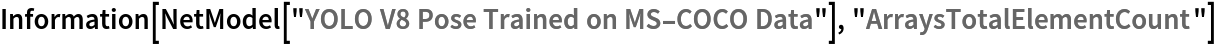Out=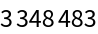Obtain the layer type counts:

 In:=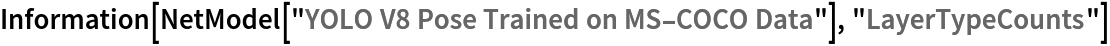Out=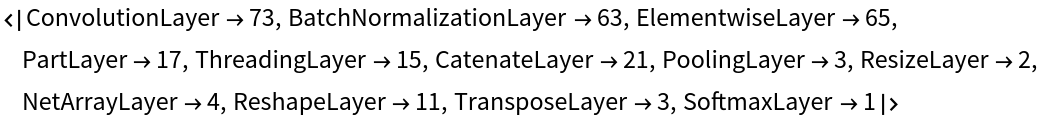Display the summary graphic:

 In:=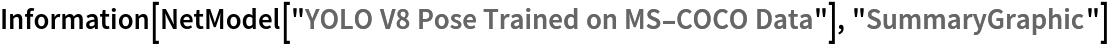Out=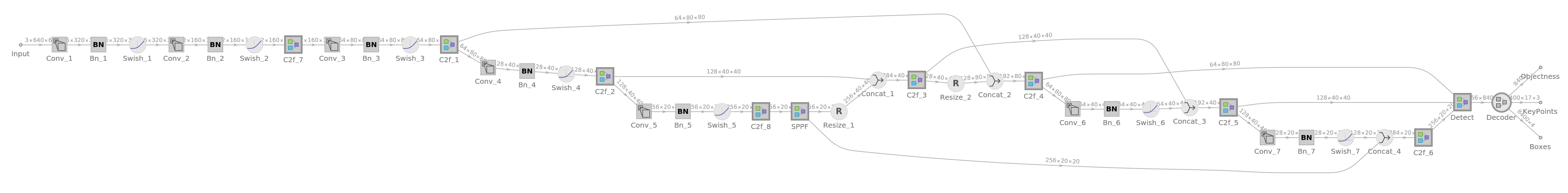### Export to ONNX

Export the net to the ONNX format:

 In:=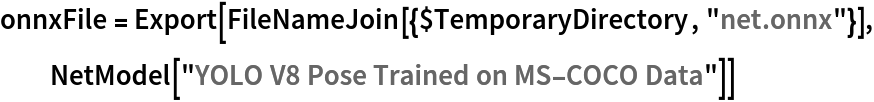Out=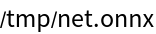Get the size of the ONNX file:

 In:=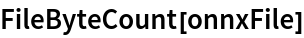Out=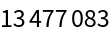The size is similar to the byte count of the resource object:

 In:=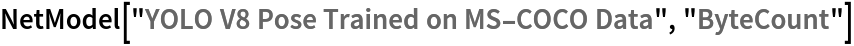Out=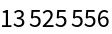Check some metadata of the ONNX model:

 In:=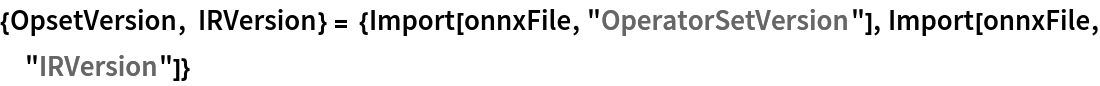Out=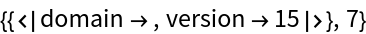Import the model back into Wolfram Language. However, the NetEncoder and NetDecoder will be absent because they are not supported by ONNX:

 In:=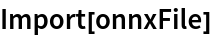Out=